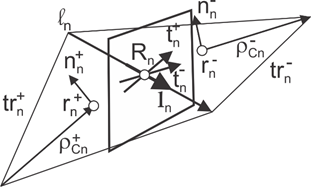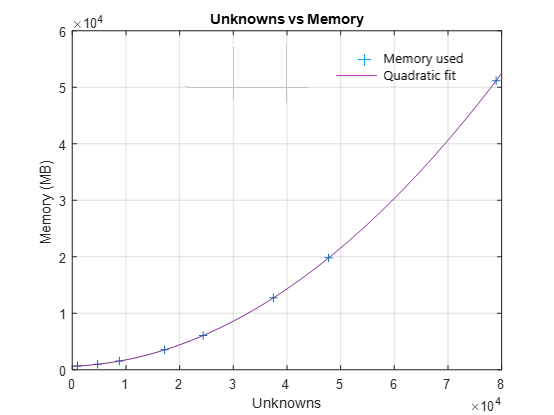## Fast Multipole Method for Large Structures

The fast multipole method (FMM) computational technique in Antenna Toolbox™ allows you to model and analyze antennas and arrays on large platforms like aircrafts and automobiles.

### Direct Solvers

The first step in the computational solution of electromagnetic problems is to discretize Maxwell's equations. The process results in this matrix-vector system:

`$V=ZI$`
• V — Applied voltage vector. This signal can be voltage or power applied to the antenna or an incident signal falling on the antenna.

• I — Current vector that represents current on the antenna surface.

• Z — Interaction matrix or impedance matrix that relates V to I.

Antenna Toolbox uses Method of Moments Solver for Metal and Dielectric Structures to calculate the interaction matrix and solve system equations.

To calculate the surface currents on the antenna structure, you first define Rao-Wilton-Glisson (RWG) basis functions. The RWG basis function is a pair of triangles that share an edge, and is shown in the figure.For any two triangle patches, ${t}_{n}^{+}$ and ${t}_{n}^{-}$, having areas ${A}_{n}^{+}$ and ${A}_{n}^{-}$, and sharing common edge ${l}_{n}$, the basis function is

`$\begin{array}{l}{\stackrel{\to }{f}}_{n}\left(\stackrel{\to }{r}\right)=\left\{\begin{array}{cc}\frac{{l}_{n}}{2{A}_{n}^{+}}{\stackrel{\to }{\rho }}_{n}^{+S},& \stackrel{\to }{r}\text{\hspace{0.17em}}\in \text{\hspace{0.17em}}{t}_{n}^{+}\\ \frac{{l}_{n}}{2{A}_{n}^{-}}{\stackrel{\to }{\rho }}_{n}^{-S},& \stackrel{\to }{r}\text{\hspace{0.17em}}\in \text{\hspace{0.17em}}{t}_{n}^{-}\end{array}\text{ }\\ \end{array}$`
• ${\stackrel{\to }{\rho }}_{n}^{+}=\stackrel{\to }{r}-{\stackrel{\to }{r}}_{n}^{+}$ — Vector drawn from the free vertex of triangle ${t}_{n}^{+}$ to observation point $\stackrel{\to }{r}$

• ${\stackrel{\to }{\rho }}_{n}^{-}={\stackrel{\to }{r}}_{n}^{+}-\stackrel{\to }{r}$ — Vector drawn from the observation point to the free vertex of the triangle ${t}_{n}^{-}$

and

`$\nabla \cdot {\stackrel{\to }{f}}_{n}\left(\stackrel{\to }{r}\right)=\left\{\begin{array}{cc}\frac{{l}_{n}}{{A}_{n}^{+}},& \stackrel{\to }{r}\text{\hspace{0.17em}}\in \text{\hspace{0.17em}}{t}_{n}^{+}\\ -\frac{{l}_{n}}{{A}_{n}^{-}},& \stackrel{\to }{r}\text{\hspace{0.17em}}\in \text{\hspace{0.17em}}{t}_{n}^{-}\end{array}$`

The basis function is zero outside the two adjacent triangles ${t}_{n}^{+}$ and ${t}_{n}^{-}$. The RWG vector basis function is linear and has no flux (no normal component) through its boundary.

### Relation Between Memory Used and Problem Size

The interaction matrix Z is a complex dense symmetric matrix. It is a square N-by-N matrix, where N is the number of basis functions, that is, the number of interior edges in the structure. Consider the scenario of a large structure like an aircraft or a ship. Typical narrow-band antennas like the dipole or patch are half-wavelength in size, but ships or aircraft can often be at least 100 wavelengths or more in size. To solve for the electromagnetic effects of either radiation or scattering from this structure using a full-wave solver, the first step is to mesh the structure and then form the basis functions. Doing so generates more than 50,000 triangles. Since the memory requirement for the direct solver is of the order of O(N2), in basis function space, the growth is as shown in this plot.Under any of the following conditions the number of the unknowns become very large:

• High analysis frequency

• Structure refined with a finer mesh

• Analysis of a physically large structure

### Fast Multipole Method (FMM)

The acceleration achieved by the FMM algorithm is due to its ability to subdivide the problem into successively smaller spatial regions, thereby ensuring that the interaction between a given pair of source and target clusters are distant enough for it to be computed using multipole expansions. The following figure illustrates that.This approach fits well with our need to accelerate the computation of interactions between separated pairs of basis functions, that is source and target dipole pairs. The problem of determining the electromagnetic potential at a given set of target points in a Helmholtz type of problem can be expressed:

$u\left(r\right)=\sum _{n=1}^{N}{c}_{n}\frac{\mathrm{exp}\left(jk|r-{r}_{n}|\right)}{|r-{r}_{n}|}-{v}_{n}·\nabla \left(\frac{\mathrm{exp}\left(jk|r-{r}_{n}|\right)}{|r-{r}_{n}|}\right)$

wherein, cn and vn represent the collection of charge and dipole strengths, respectively, k is the wavenumber, and u(r) is the potential computed by FMM in 3-D space.

FMM speeds up the computation of the matrix-vector product by substantially accelerating the computation of point-to-point interactions mediated by the Green's function. The original current and charge distributions on the surface of the target are determined by introducing these coefficients back into the basis function expansion. Scattered or radiated field of the target including its radar cross-sections is then found by computing the radiation of the known surface currents and charges at required points in space. The iterative approach to determining a matrix inverse is a well-studied and established field of applied linear algebra. Among the variety of iterative solvers that exist, the generalized minimum residual (GMRES) method is a well-known technique. The Antenna toolbox uses this iterative solver.

#### Electric Field Integral Equation (EFIE)

The direct solver implemented in the Antenna Toolbox is based on electric field integral equation (EFIE). EFIE uses the electric field relationships on the surface of a metal and at any point in free space to set up the system of equations.

`${E}_{t}^{s}=-{E}_{t}^{i}$`

`${E}^{s}\left(r\right)=-j\omega A-\nabla \phi$`

The index t in the first of the two equations is used to describe the tangential component of the electric field on a metal surface, index s describes the scattered field, and index i denotes the incident field. In the second equation the relationship of the scattered field is shown in terms of the electric scalar potential φ and magnetic vector potential A.

Applying the Galerkin approach, where the test using the basis functions leads to the following key equation:

`$j\omega \left\{\frac{{l}_{m}}{2}{p}_{m}^{+}\left({r}_{m}^{+}\right)·A\left({r}_{m}^{+}\right)+\frac{{l}_{m}}{2}{p}_{m}^{-}\left({r}_{m}^{-}\right)·A\left({r}_{m}^{-}\right)\right\}-\left\{{l}_{m}\phi \left({r}_{m}^{+}\right)-{l}_{m}\phi \left({r}_{m}^{-}\right)\right\}={V}_{m}$`

`${V}_{m}=\frac{{l}_{m}}{2}{p}_{m}^{+}\left({r}_{m}^{+}\right)·{E}^{i}\left({r}_{m}^{+}\right)+\frac{{l}_{m}}{2}{p}_{m}^{-}\left({r}_{m}^{-}\right)·{E}^{i}\left({r}_{m}^{-}\right)$`

#### Magnetic Field Integral Equation (MFIE)

MFIE equation expresses the surface current density J(r) developed on the body of a metallic object in response to a magnetic field excitation. An important observation here is that the second term of MFIE is the exact physical optics (PO) approximation. This equation captures the first order solution as the PO approximation while the second term involving the integral captures the full-wave effects, thus providing a complete solution.

MFIE can be applied only to closed structures such as boxes, spheres, closed shells of aircraft, and so on. It cannot be applied, for example, to a strip dipole or monopole antenna.

`$J\left(r\right)=2n\left(r\right)×\underset{s}{\int }J\left(r\text{'}\right)×{\nabla }_{r\text{'}}\frac{\mathrm{exp}\left(-jk|r-r\text{'}|\right)}{4\pi |r-r\text{'}|}dr\text{'}+2n\left(r\right)×{H}^{i}\left(r\right)$`

Using the collocation approach leads to the equation for the MFIE implementation:

`${c}_{m}-\left\{{I}_{m}·\sum _{n=1}^{{N}_{facets}}\left(\begin{array}{c}{M}_{1}·\nabla \\ {M}_{2}·\nabla \\ {M}_{3}·\nabla \end{array}\right)\frac{\mathrm{exp}\left(-jk|{R}_{m}-{r}_{n}|\right)}{4\pi |{R}_{m}-{r}_{n}|}\right\}={I}_{m}^{PO}$`

`${M}_{1}=\left(\begin{array}{c}0\\ -{m}_{z}\\ +{m}_{y}\end{array}\right),{M}_{2}=\left(\begin{array}{c}+{m}_{z}\\ 0\\ -{m}_{x}\end{array}\right),{M}_{3}=\left(\begin{array}{c}-{m}_{y}\\ +{m}_{x}\\ 0\end{array}\right),m={I}_{n}{r}_{n}$`

#### Combined Field Integral Equation (CFIE)

CFIE uses the two equations shown for EFIE and MFIE. The term α is chosen to be 0.5 and η = 376.3Ω is the free space impedance.

`$\alpha LHS{E}_{m}+\left(1-\alpha \right)\eta LHS{H}_{m}=\alpha {V}_{m}+\left(1-\alpha \right)\eta {I}_{m}^{PO}$`

The FMM solver is applied to compute the left-hand side of this equation. LHSEm represents the left-hand side of the EFIE and LHSHm represents the left-hand side of the MFIE.

 Flatironinstitute/FMM3D. Fortran. 2018. Reprint, Flatiron Institute, 2021. https://github.com/flatironinstitute/FMM3D.

 Greengard, L, and V Rokhlin. “A Fast Algorithm for Particle Simulations.” Journal of Computational Physics 73, no. 2 (December 1987): 325–48. https://doi.org/10.1016/0021-9991(87)90140-9.

 Rius JM, Úbeda E, Parrón J. On The Testing of the Magnetic Field Integral Equation With RWG Basis Functions in Method of Moments. IEEE Trans. Antennas and Propagation, vol. AP-49, no. 11, pp. 1550-1553.

 Rao SM, Wilton DR, Glisson AW. Electromagnetic Scattering by Surfaces of Arbitrary Shape. IEEE Trans. on Antennas and Propagation. 1982 May;30(3):409-418. doi: 001 8-926X/82/0500-O409.